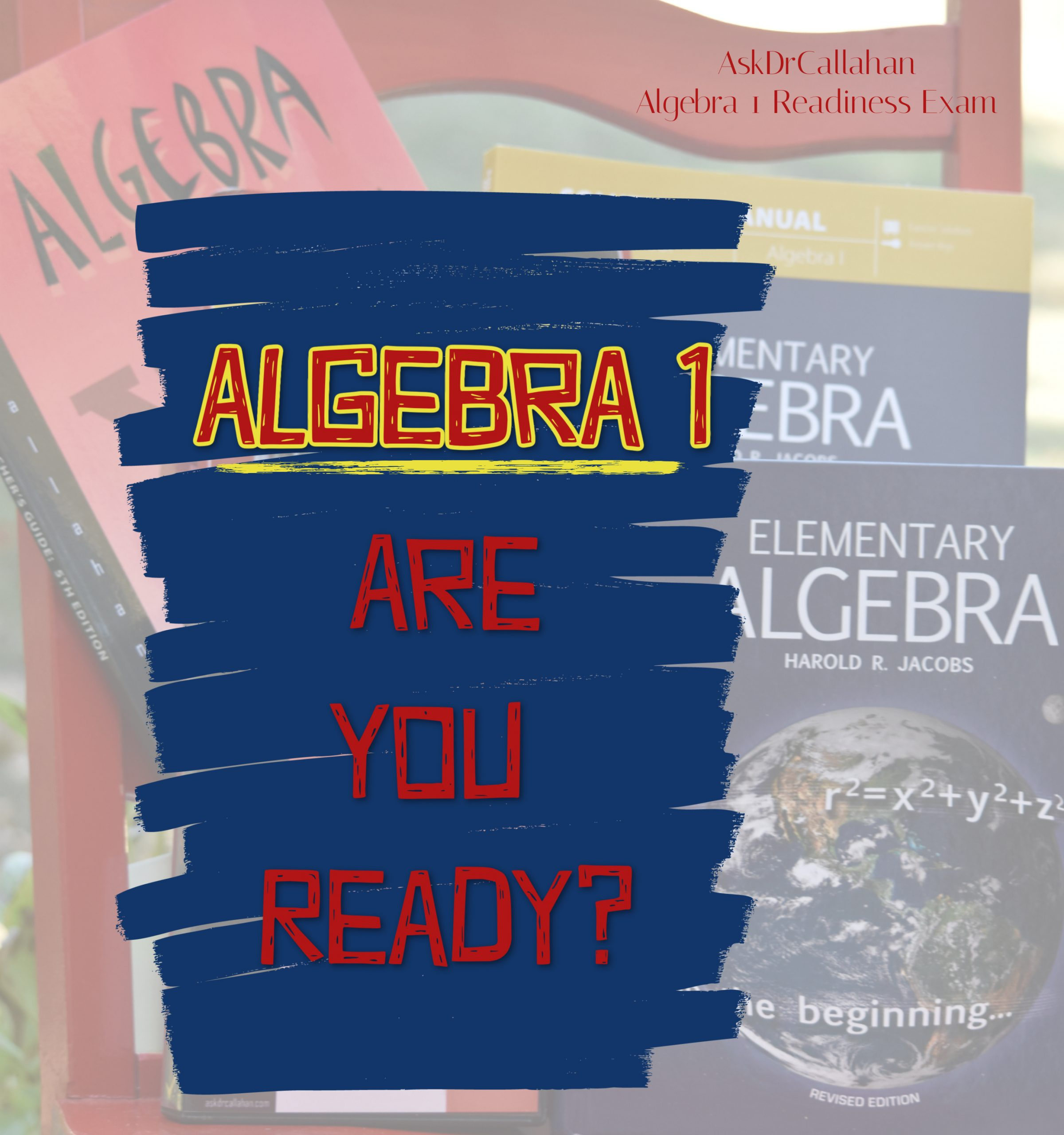Posted on

# Algebra Readiness Test | Do your students need Algebra or Prealgebra?Do you need an Algebra Readiness Test?

Many students and parents come to algebra with a bit of fear and apprehension. As parents, we are just not sure they are ready to jump into higher math like algebra and geometry. As students, they have often heard horror stories.

But the truth is, algebra is not really that bad. Many students who are bored to tears with basic math find algebra a welcome relief and, as a result, do better in math than they have done in years.

## What is Prealgebra

We have been taught that prealgebra must come before algebra. After all, that is how the school systems usually do it. But as homeschoolers, we have the luxury of deciding what is best for our children and not just following the system’s way.

Prealgebra is usually taught in the 6th or 7th grade as a preparation stage to get to algebra.

Concepts covered in prealgebra are

• negative numbers,
• integers,
• properties of operations,
• exponents,
• factorization
• basic equations.

Generally, students ready for prealgebra have a good grasp of basic concepts like addition, subtraction, multiplication, division, fractions, decimals, exponents, variables, and inequalities. This does not mean they are perfect in any of these areas – but just that they get the concepts and can do the work.

Something to think about when considering pre-algebra is that most students can head straight into algebra. Arithmetic and algebraic expressions are the same except that algebra uses variables.

For instance, in arithmetic we are taught that 1 + 1 = 2. It is a simple relationship between two numbers.

In algebra, while this relationship stays the same, you may be told that 1 + x =2 and be asked to identify that x has to equal 1 since the rules you learned back in arithmetic are still true.

The idea of using the more abstract “x” is the biggest hurdle for students. So if your student is strong in the idea of “x” already, I would consider just going ahead with Algebra I.

The biggest differences between prealgebra and algebra are the pace and length. Prealgebra introduces algebra concepts and takes each one slower and therefore does not cover as much material as a standard Algebra I course. Some parents find it is just as easy to take a regular Algebra I course and do it in two years, especially if the student is in the 6th or 7th grade. Most older students can dive straight into Algebra without pre-algebra and finish in one school year. Jacobs Algebra starts out slow and starts with the math basics and gently moves the student into the abstract critical thinking of algebra.

## Jacobs Algebra Contains Prealgebra

Our algebra course is based on Harold Jacobs’s textbook Elementary Algebra. This course is really a prealgebra and algebra combined. It starts with basic concepts you would find in a prealgebra course and then slowly moves into algebraic methods. If your student needs more time, it is quite easy to split the book in half, take one half of the book in one year, and complete the second half in the second year. In this way, students are given the slower pace of a prealgebra course, allowed the opportunity to be introduced to the idea of algebra without running headlong into a complete course in one single year. Plus, parents only have to buy one curriculum, and it lasts them through two years of math. Harold Jacobs’s text is particularly a good choice for this option because it starts with some review, so students are eased into Algebra I without being overwhelmed. Again, don’t underestimate your student either, they may easily move through the Algebra book in one year as well.

We offer this textbook in our Algebra course along with video instruction and a free year of support (or two years if you choose that route) to our customers so that taking this slower pace is easier on the parents and the student.

It is sometimes hard to assess whether your student needs the slower pace of prealgebra or if going straight into algebra would be better. To help you with this step of the assessment, we recommend an algebra readiness exam.